Insitute of Mathematical Science

Colloquium: L_1 ISOPERIMETRIC INEQUALITY ON THE UNIT DISK

Colloquium| Institute of Mathematical Sciences

Time：Wednesday, September 22nd, 2021, 16:00-17:00

LocationR408, IMS

Speaker: Meijun Zhu, University of Oklahoma

Abstract: We show: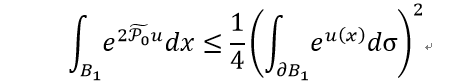holds for allwhere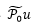is the harmonic extension ofto the unit ball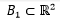. Moreover, the equality holds if and only if u=c or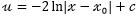where c is a constant and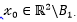. The above inequality was proved in 1921 by Carleman for all analytic functions on B1 (thus gave an analytic proof of isoperimetric inequality on simply connected domains). This is a joint work with Jingbo Dou.

200031（岳阳路校区）